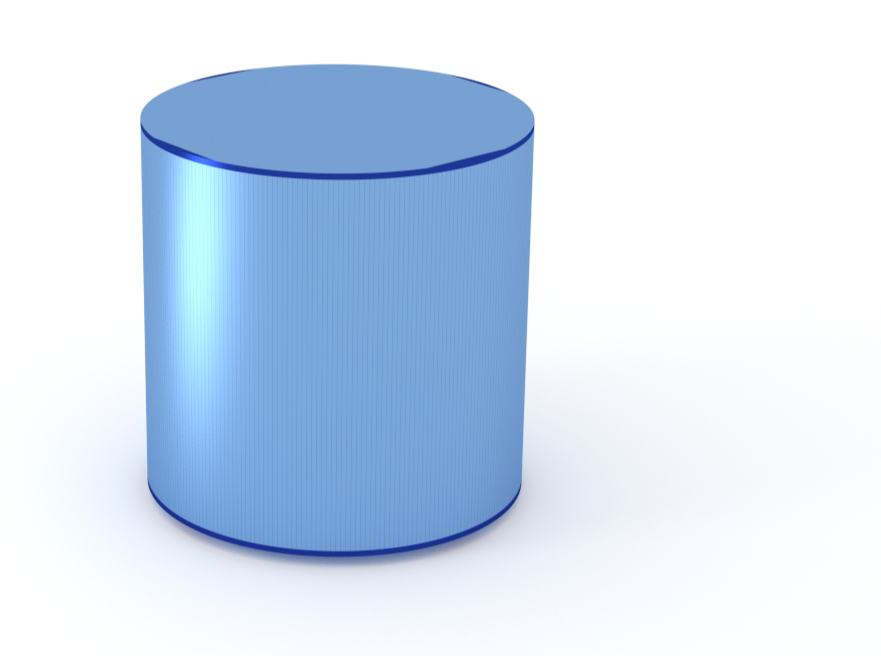### Cylinder

An upright circular cylinder.#### Constructor

Cylinder(R, H)


Parameters:

• H, height

#### Usage

As for any other Form factor.

#### Implementation

Class Cylinder inherits from the interface class IFormFactor .

Form factor is computed as

$$F(\mathbf{q})=2\pi R^2H \space \exp\Big(iq_z\dfrac{H}{2}\Big) \text{sinc}\Big(q_z\dfrac{H}{2}\Big) \dfrac{J_1(q_{\parallel}R)}{q_{\parallel}R} ,$$ with the notation $$q_{||} := \sqrt{q_x^2 + q_y^2}.$$

Volume has been validated against $$V=\pi R^2H.$$

More general:

#### Example

Scattering by uncorrelated, oriented cylinders for horizontal incidence. Rotation around $y$ axis:Generated by Examples/ff/Cylinder.py .

#### History

This form factor is well known; it goes back to Lord Rayleigh.

In IsGISAXS and FitGISAXS, it has been implemented as form factor “Cylinder” [manual, Eq. 2.26; Renaud 2009, Eq. 223; Babonneau 2013].